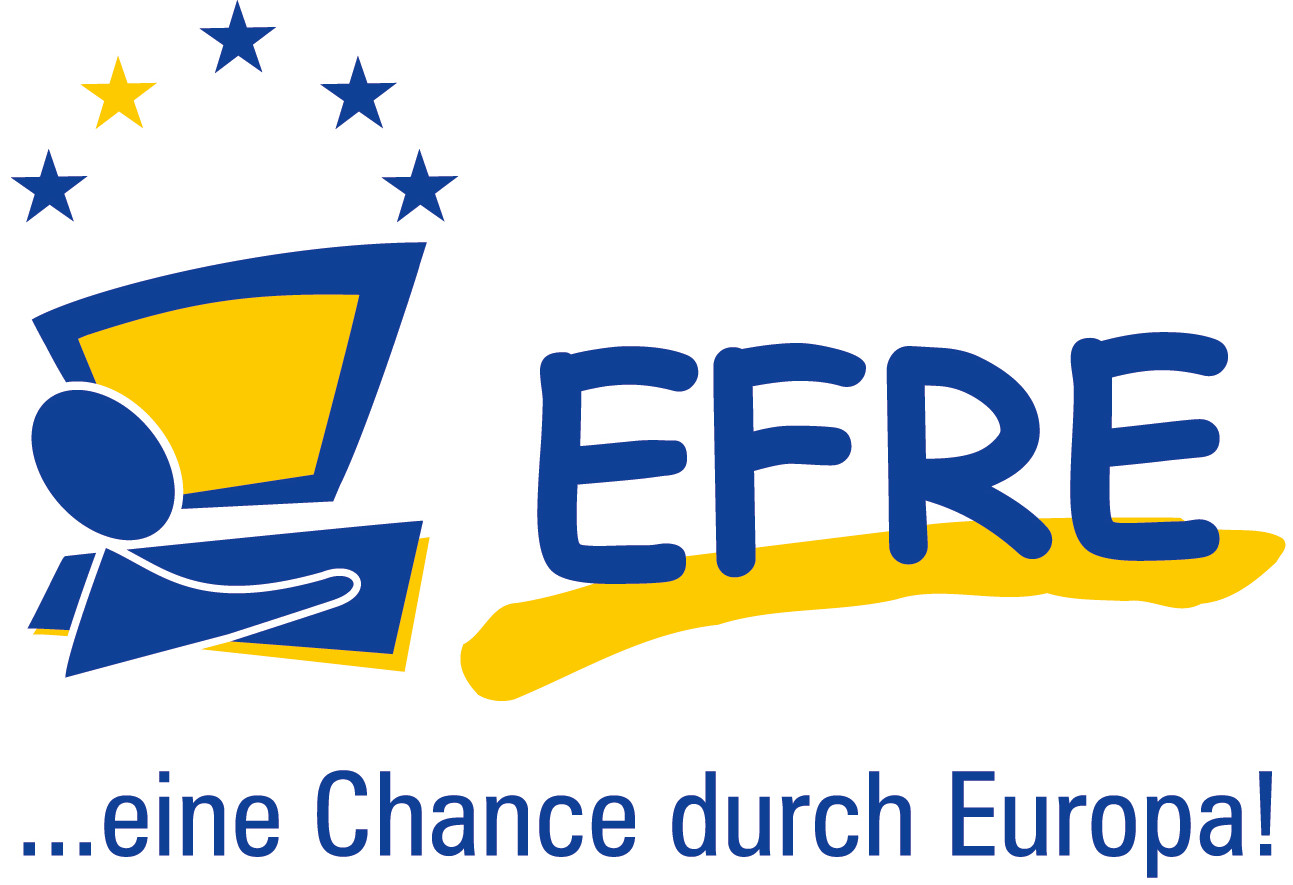# Model calibration in environmental simulations (MUSI)

The project "MUSI" - "Model Calibration in Environmental Simulations" is a cooperation between WIAS Berlin and DHI-WASY GmbH supported by the Investionsbank Berlin (IBB) and the European Regional Development Fund . It runs from 2013-2016.

Mathematical modeling and numerical simulation of flow and transport in subsurface structures is an indispensible tool hydrological engineering, environmental protection and other fields. The starting point of these simulation efforts is the mathematical description of stationary groundwater flow in an aquifer. It can be described by the so called Darcy equation $$- \vec q(\vec x) = \kappa(\vec x) \nabla u(\vec x)$$ which relates the flow velocity $\vec q$ to the gradient of the hydraulic head $u$ multiplied by the coefficient $\kappa$ describing the permeability of the subsurface structure, together with a continuity equation $$-\nabla \cdot \vec q(\vec x) = f(\vec x)$$ balancing the flux vector with sources and sinks described by $f$. $\vec x$ is the space coordinate. Given proper boundary conditions and values of $\kappa$ and $f$, the existence of a unique solution $u(\vec x)$ of this problem can be proven. However, as a rule, no mathematical formula for $u(\vec x)$ can be found.

The finite element discretization method allows to define an approximate problem wich can be solved by an algorithm on a computer, yielding an approximate solution $u_h(\vec x)$ . However, even in this case, precise information about the spatial distribution of $\kappa(\vec x)$ is required. Often, this information is available in a less precise manner. The function $\kappa(\vec x)$ may in fact be a assumed to attain random values within certain bounds. Each realization of the random $\kappa$ yields another solution $u_h$. The uncertainty in $\kappa$ is thus propagated to the uncertainty of $u_h$, allowing to assess the accuracy of the solution depending on the amount of effort put into obtaining precise values of $\kappa$.

The project aims at assessing modern methods which allow to cope with this uncertainty quantification problem in an efficient way. These include multilevel Monte-Carlo methods and stochastic collocation methods in hierarchical and adaptive variants.

To solve the inverse problem -- obtaining information on $\kappa(x)$ from measurements of $u(x)$ in certain points -- approaches to stochastic Bayesian inversion like Markov Chain Monte Carlo methods and Ensemble Kalman Filter methods are investigated.

Accumulation and assessment of this knowledge are aimed at fostering the competitive advantage of the hydrological simulation software developed and marketed by the project partner DHI-WASY GmbH.

Contact: Dr. Jürgen Fuhrmann